## It is common that Governments revise travel fares from time to time based on various factors such as inflation ( a general increase in prices and fall in the purchasing value of money) on different types of vehicles like auto, Rickshaws, taxis, Radio cab etc. The auto charges in a city comprise of a fixed charge together with the charge for the distance covered. Study the following situations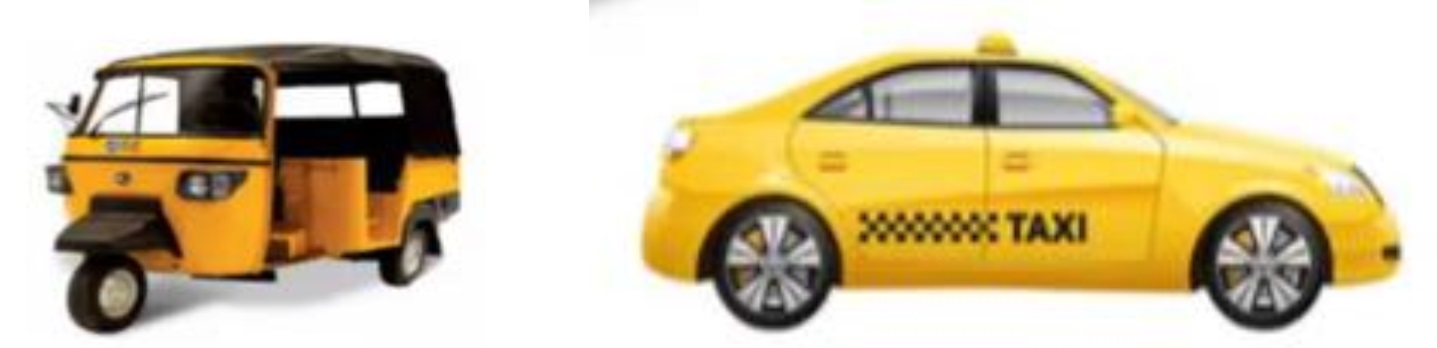Name of the city Distance travelled (Km) Amount paid (Rs.) City A 10 75 15 110 City B 8 91 14 145

## Situation 1: In city A, for a journey of 10 km, the charge paid is Rs 75 and for a journey of 15 km, the charge paid is Rs 110. Situation 2: In a city B, for a journey of 8km, the charge paid is Rs 91 and for a journey of 14 km, the charge paid is Rs 145.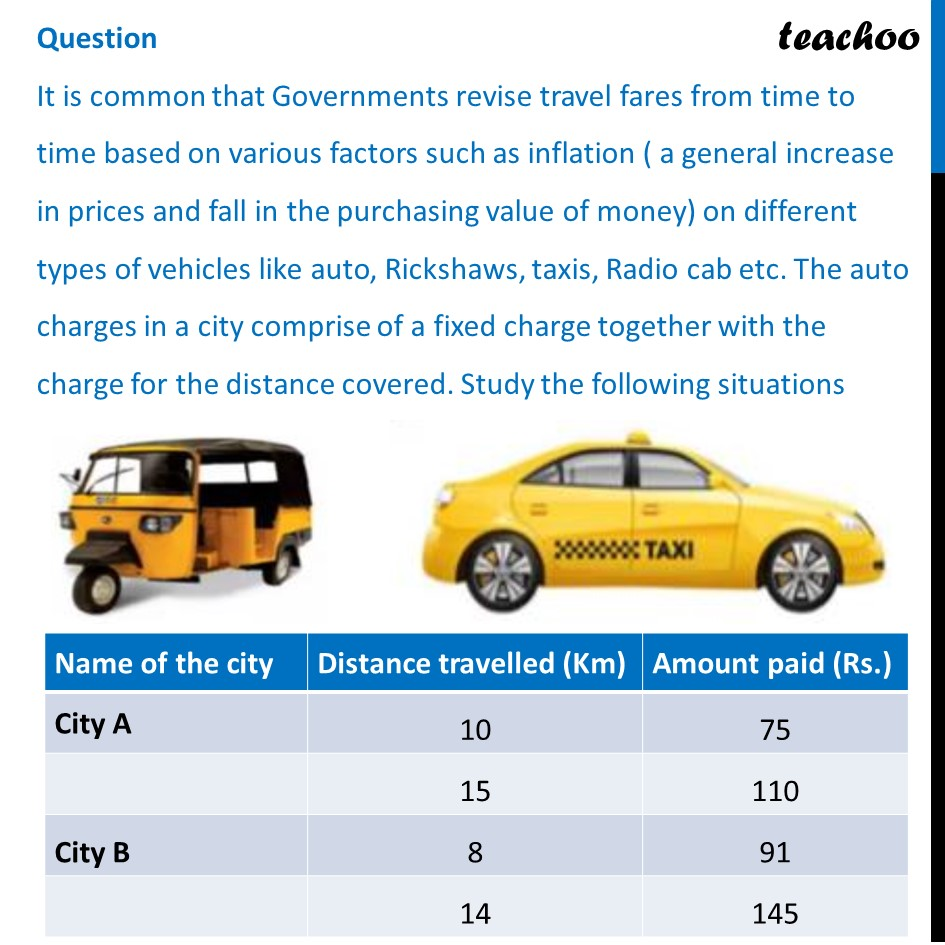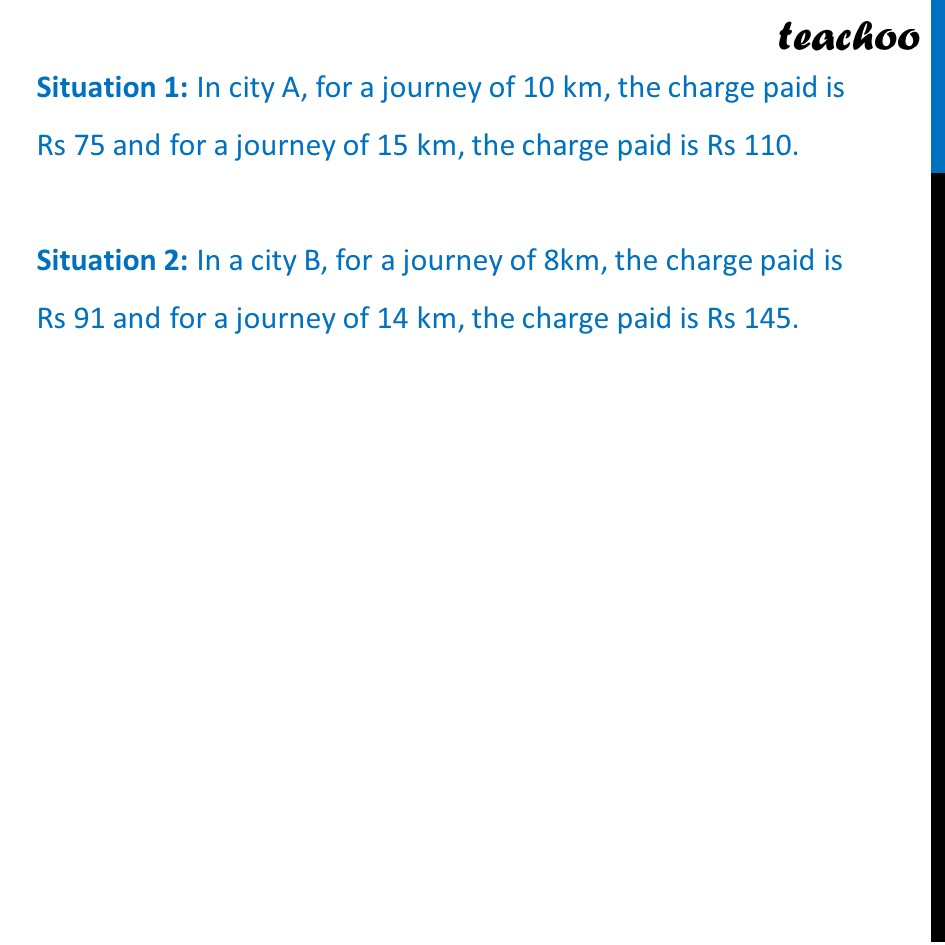## If the fixed charges of auto rickshaw be Rs x and the running charges be Rs y km/hr, the pair of linear equations representing situation is (a) x + 10y  = 110, x + 15y = 75 (b) x + 10y = 75, x + 15y = 110 (c) 10x + y = 110, 15x + y = 75 (d) 10x + y = 75, 15x + y =110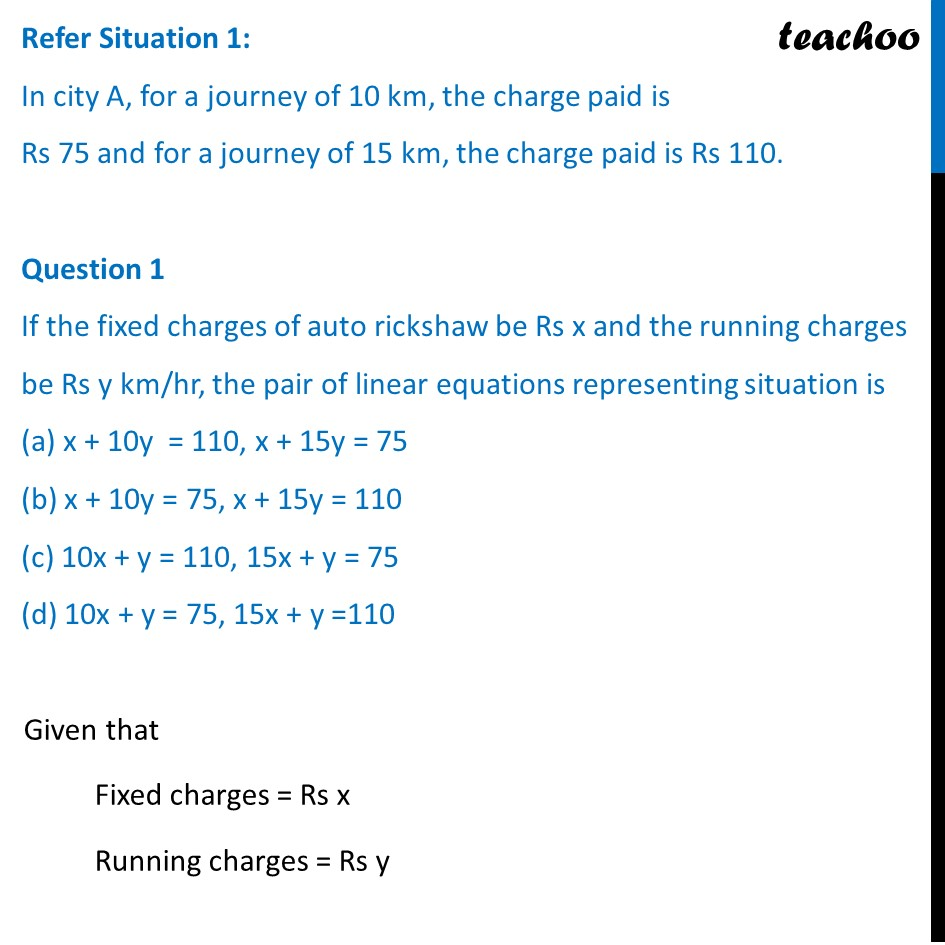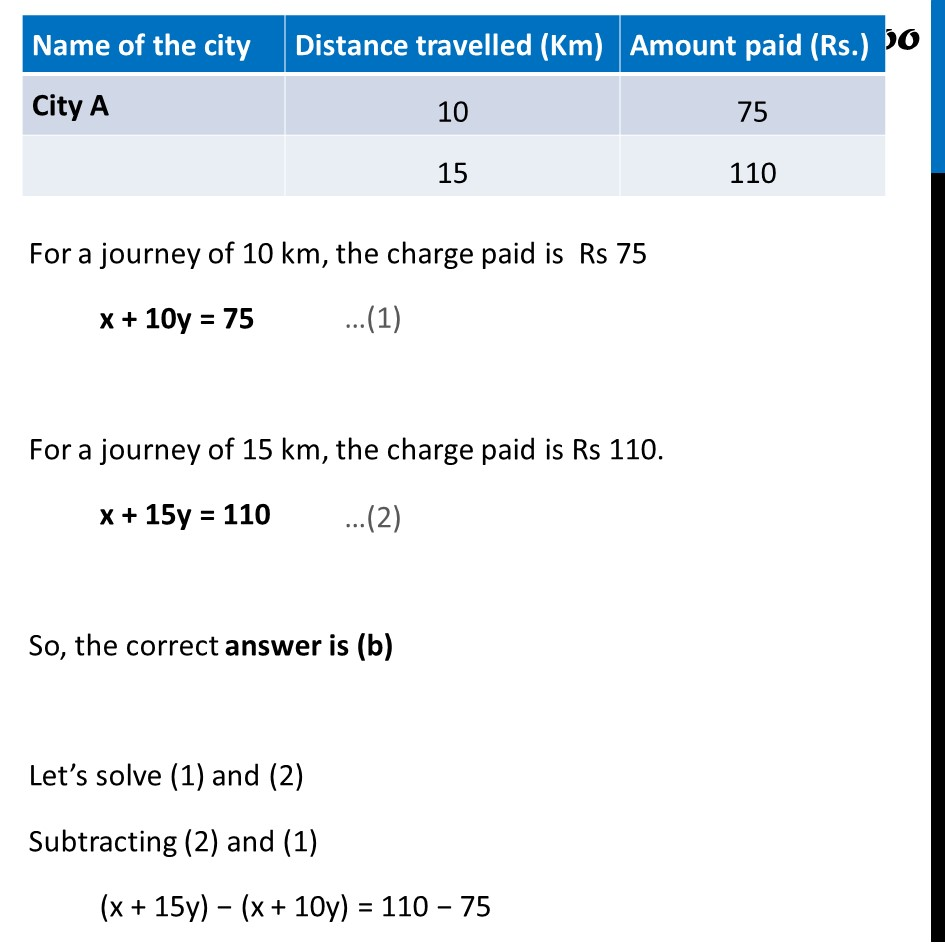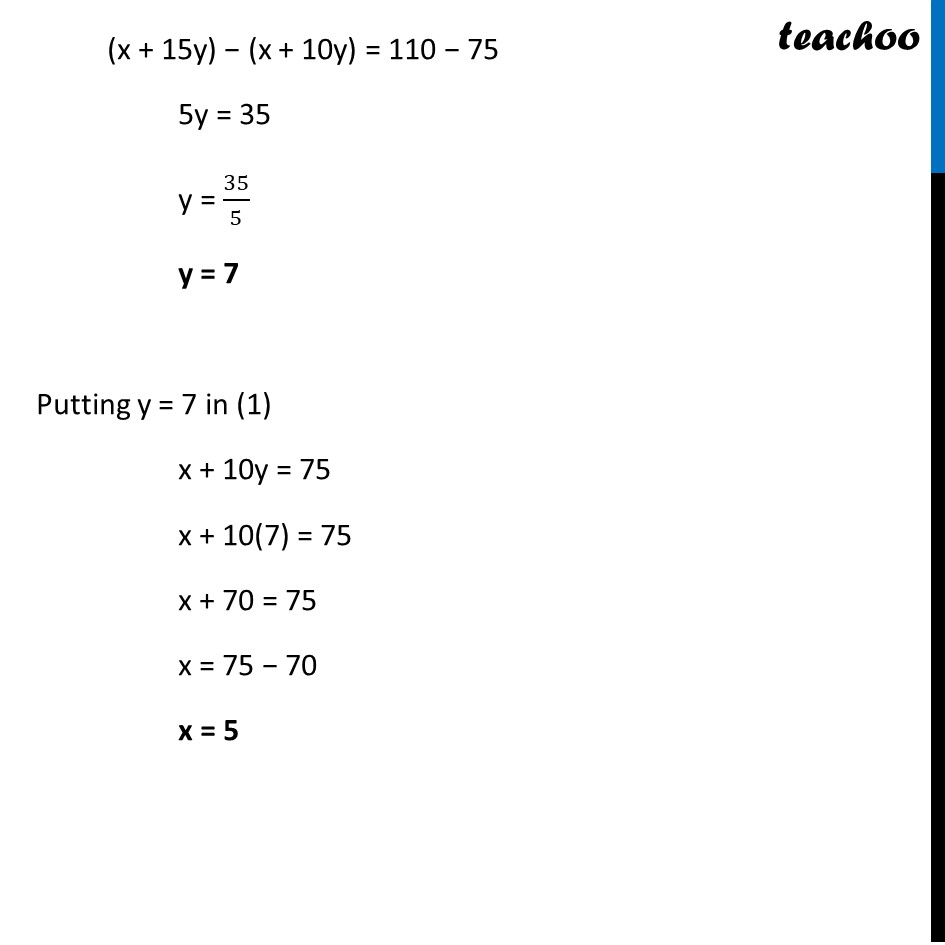## A person travels a distance of 50 km. The amount he has to pay is (a) Rs 155 (b) Rs 255 (c) Rs 355 (d) Rs 455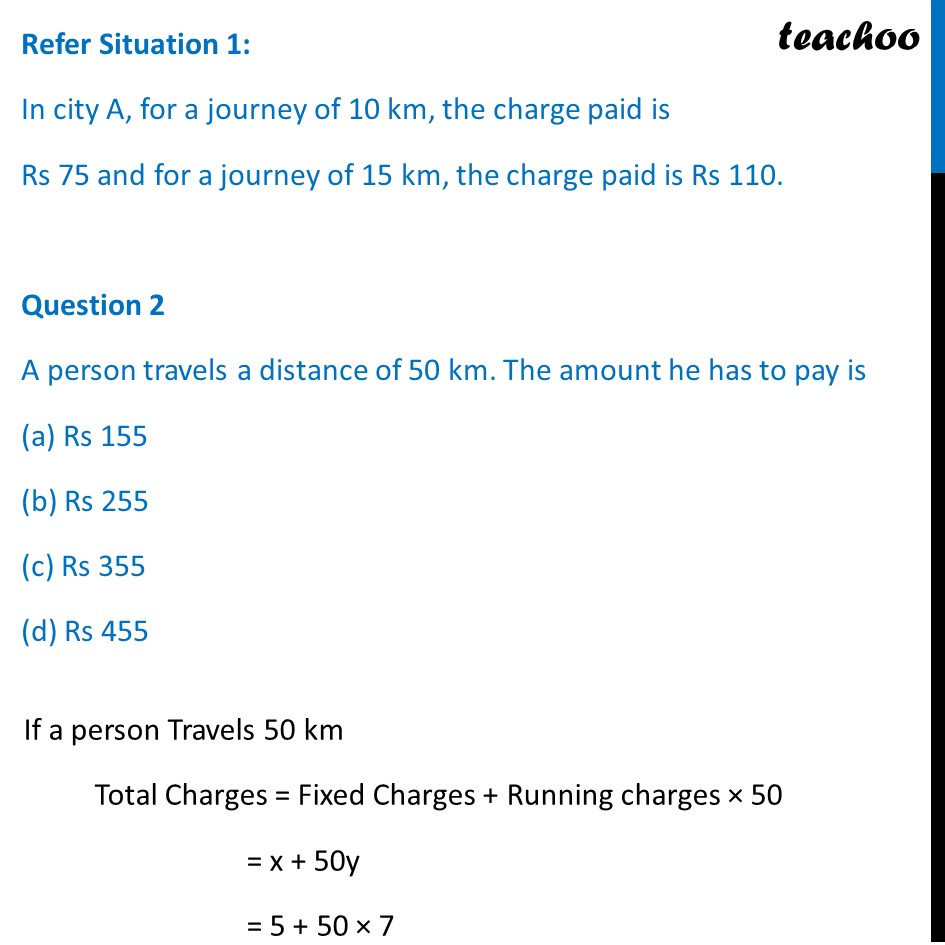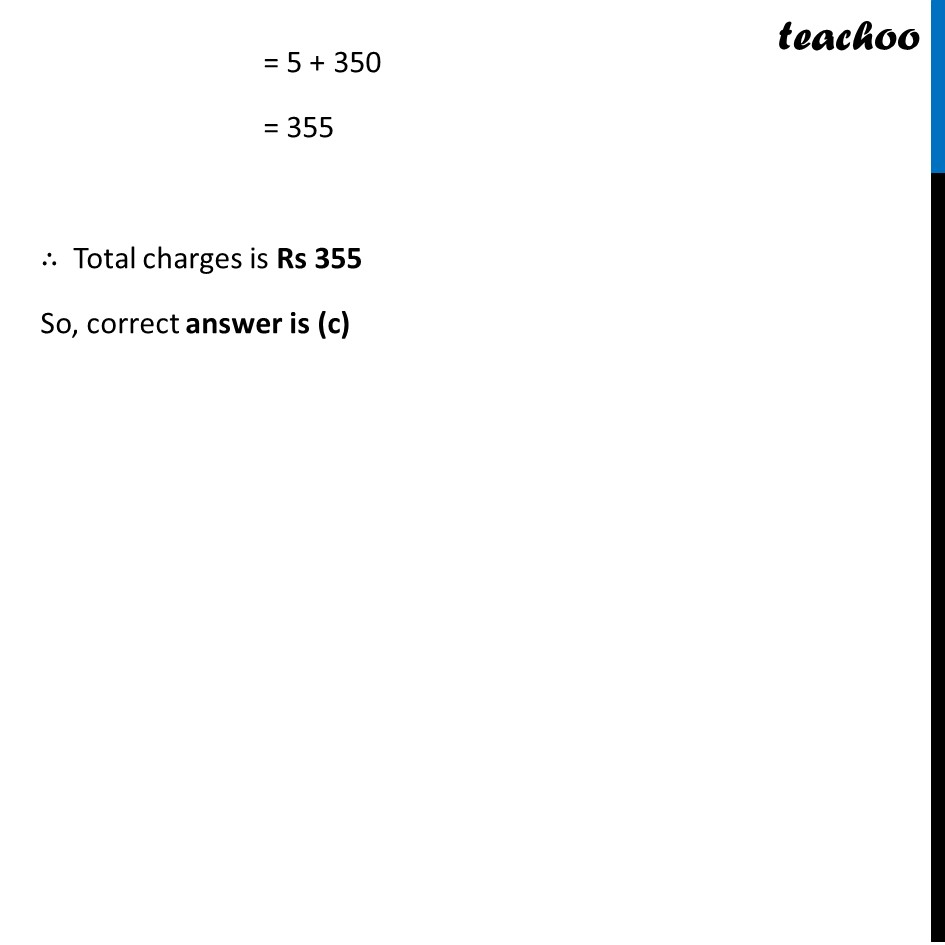## What will a person have to pay for travelling a distance of 30 km? (a) Rs 185 (b) Rs 289 (c) Rs 275 (d) Rs 305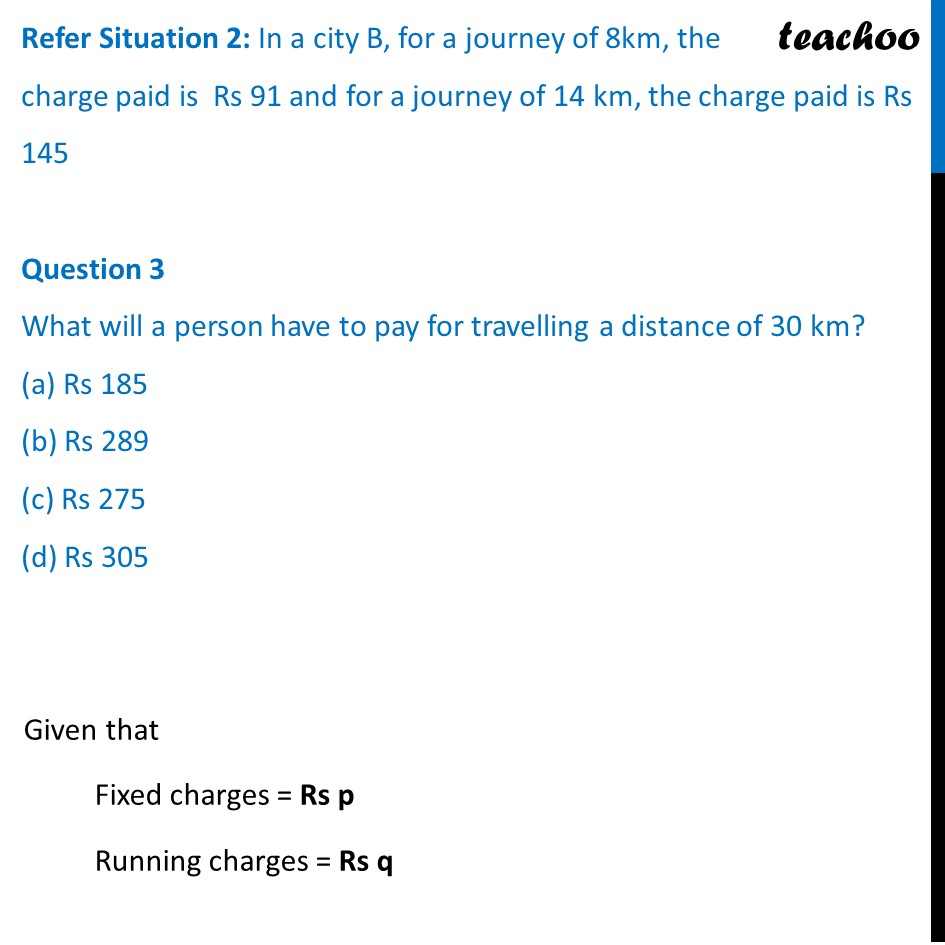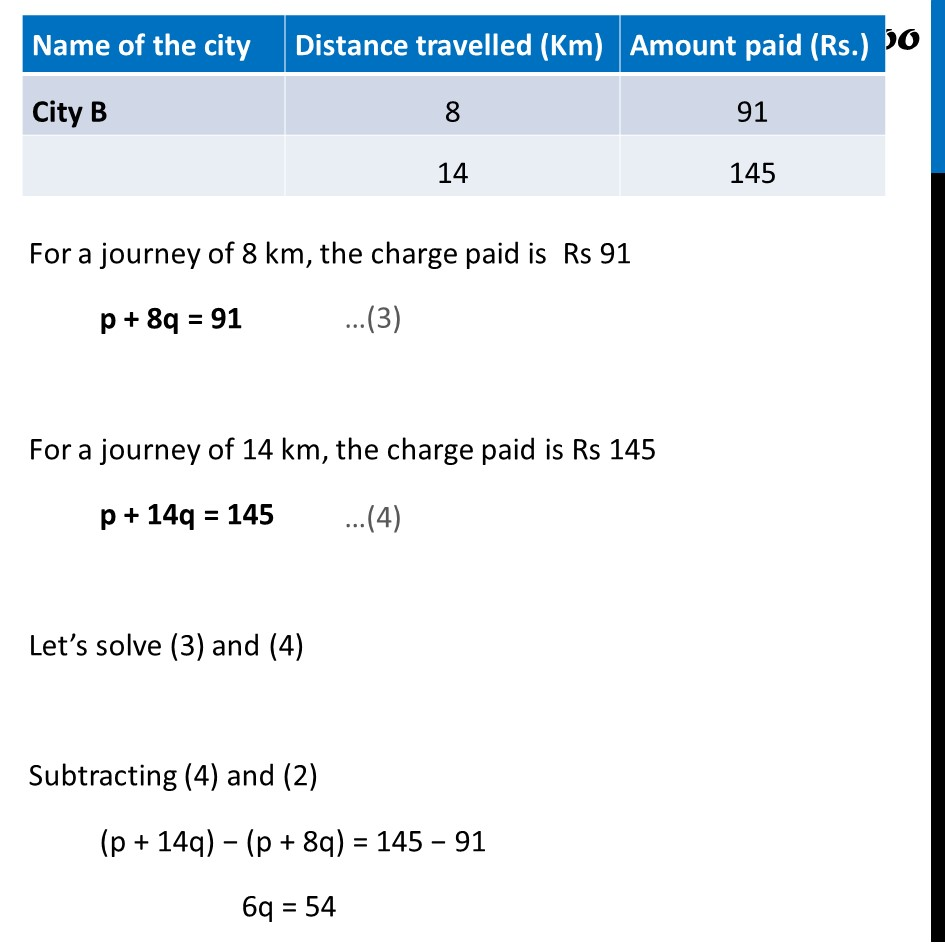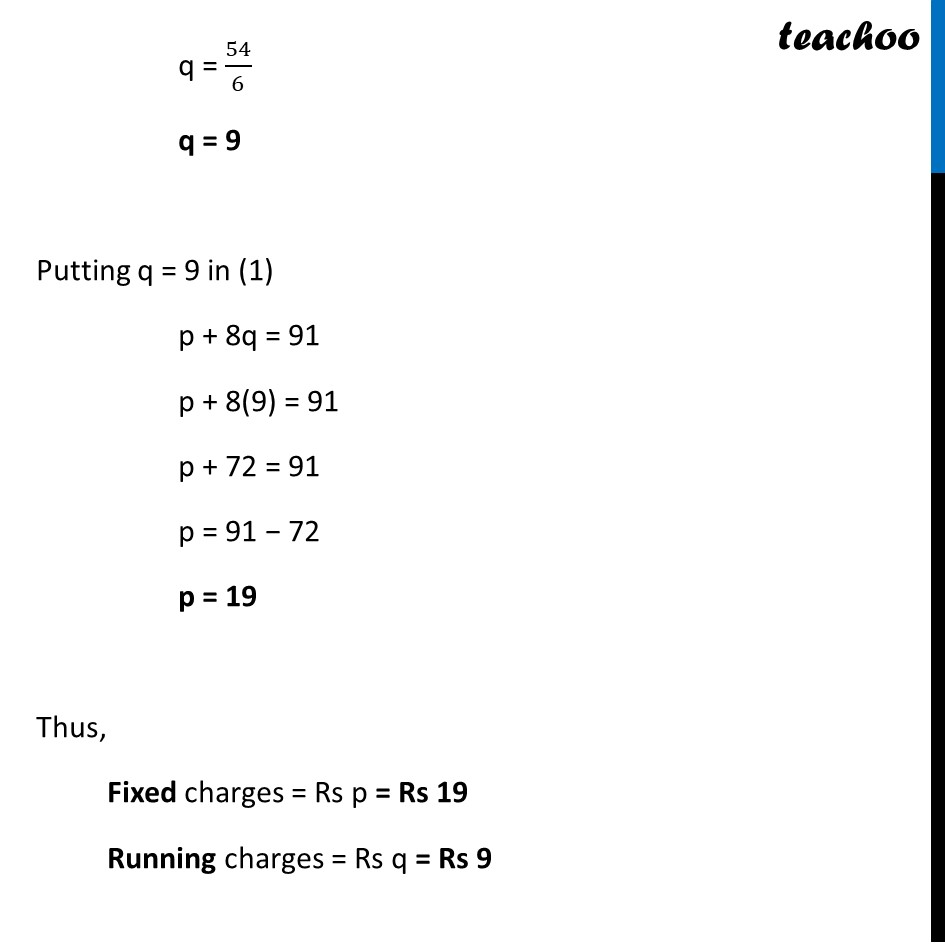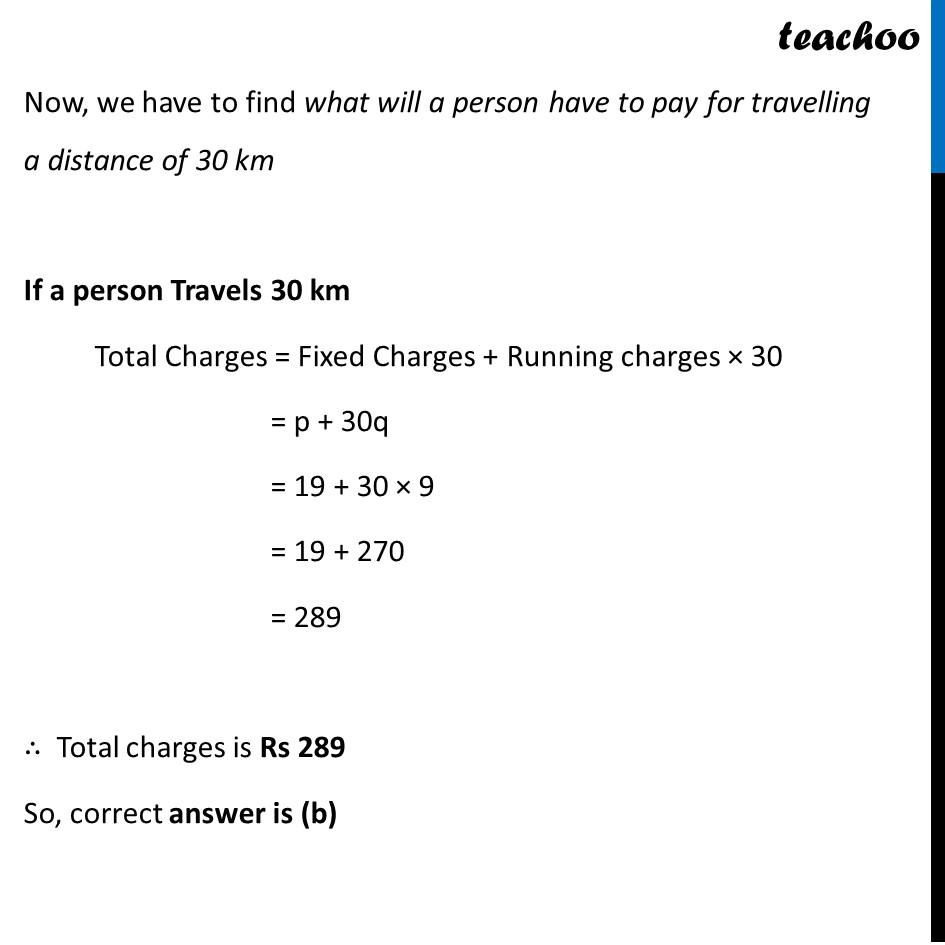## The graph of lines representing the conditions are: (situation 2)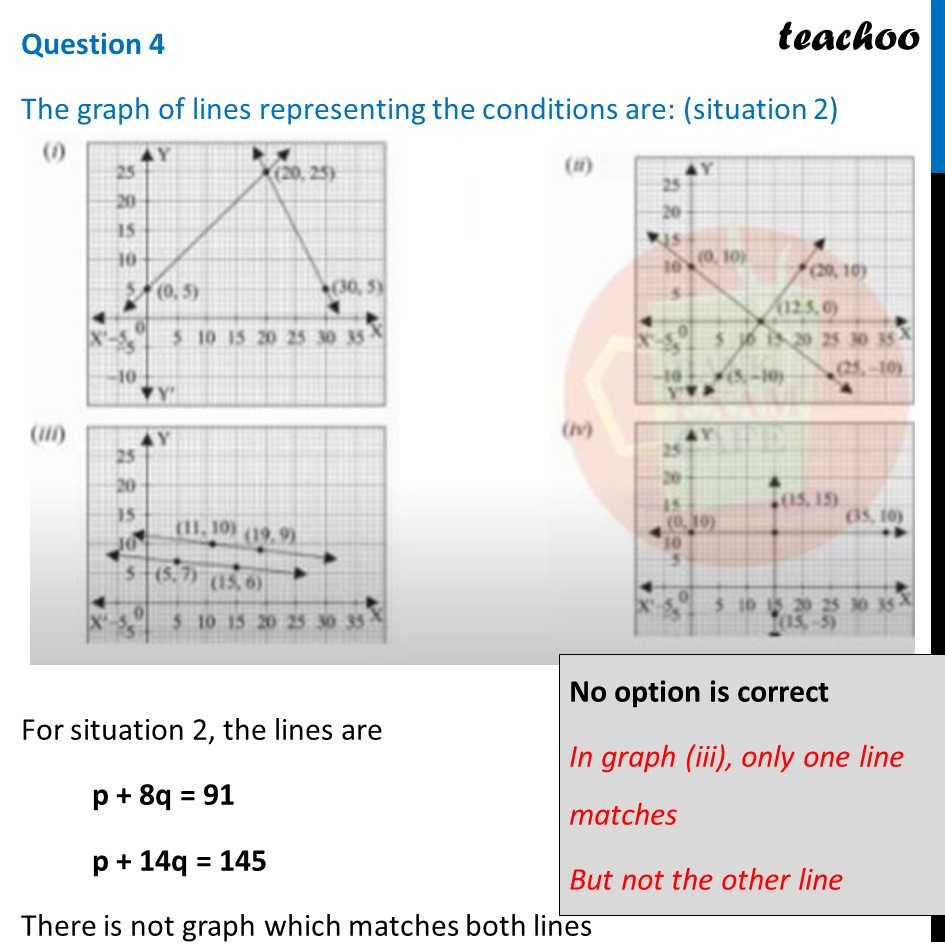1. Chapter 3 Class 10 Pair of Linear Equations in Two Variables (Term 1)
2. Serial order wise
3. Case Based Questions (MCQ)

Transcript

Question It is common that Governments revise travel fares from time to time based on various factors such as inflation ( a general increase in prices and fall in the purchasing value of money) on different types of vehicles like auto, Rickshaws, taxis, Radio cab etc. The auto charges in a city comprise of a fixed charge together with the charge for the distance covered. Study the following situations Situation 1: In city A, for a journey of 10 km, the charge paid is Rs 75 and for a journey of 15 km, the charge paid is Rs 110. Situation 2: In a city B, for a journey of 8km, the charge paid is Rs 91 and for a journey of 14 km, the charge paid is Rs 145.Refer Situation 1: In city A, for a journey of 10 km, the charge paid is Rs 75 and for a journey of 15 km, the charge paid is Rs 110. Question 1 If the fixed charges of auto rickshaw be Rs x and the running charges be Rs y km/hr, the pair of linear equations representing situation is (a) x + 10y = 110, x + 15y = 75 (b) x + 10y = 75, x + 15y = 110 (c) 10x + y = 110, 15x + y = 75 (d) 10x + y = 75, 15x + y =110 Given that Fixed charges = Rs x Running charges = Rs y For a journey of 10 km, the charge paid is Rs 75 x + 10y = 75 For a journey of 15 km, the charge paid is Rs 110. x + 15y = 110 So, the correct answer is (b) Let’s solve (1) and (2) Subtracting (2) and (1) (x + 15y) − (x + 10y) = 110 − 75 (x + 15y) − (x + 10y) = 110 − 75 5y = 35 y = 35/5 y = 7 Putting y = 7 in (1) x + 10y = 75 x + 10(7) = 75 x + 70 = 75 x = 75 − 70 x = 5 Refer Situation 1: In city A, for a journey of 10 km, the charge paid is Rs 75 and for a journey of 15 km, the charge paid is Rs 110. Question 2 A person travels a distance of 50 km. The amount he has to pay is (a) Rs 155 (b) Rs 255 (c) Rs 355 (d) Rs 455 If a person Travels 50 km Total Charges = Fixed Charges + Running charges × 50 = x + 50y = 5 + 50 × 7 = 5 + 350 = 355 ∴ Total charges is Rs 355 So, correct answer is (c) Refer Situation 2: In a city B, for a journey of 8km, the charge paid is Rs 91 and for a journey of 14 km, the charge paid is Rs 145 Question 3 What will a person have to pay for travelling a distance of 30 km? (a) Rs 185 (b) Rs 289 (c) Rs 275 (d) Rs 305 Given that Fixed charges = Rs p Running charges = Rs q For a journey of 8 km, the charge paid is Rs 91 p + 8q = 91 For a journey of 14 km, the charge paid is Rs 145 p + 14q = 145 Let’s solve (3) and (4) Subtracting (4) and (2) (p + 14q) − (p + 8q) = 145 − 91 6q = 54 q = 54/6 q = 9 Putting q = 9 in (1) p + 8q = 91 p + 8(9) = 91 p + 72 = 91 p = 91 − 72 p = 19 Thus, Fixed charges = Rs p = Rs 19 Running charges = Rs q = Rs 9 Now, we have to find what will a person have to pay for travelling a distance of 30 km If a person Travels 30 km Total Charges = Fixed Charges + Running charges × 30 = p + 30q = 19 + 30 × 9 = 19 + 270 = 289 ∴ Total charges is Rs 289 So, correct answer is (b) Question 4 The graph of lines representing the conditions are: (situation 2) For situation 2, the lines are p + 8q = 91 p + 14q = 145 There is not graph which matches both lines No option is correct In graph (iii), only one line matches But not the other line

Case Based Questions (MCQ)